Download Presentation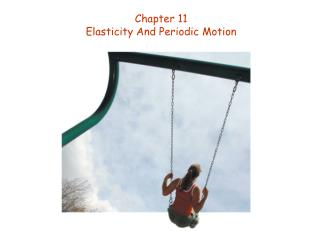Chapter 11 Elasticity And Periodic Motion

# Chapter 11 Elasticity And Periodic Motion - PowerPoint PPT Presentation

Chapter 11 Elasticity And Periodic Motion. Stress characterizes the strength of the force associated with the stretch, squeeze, or twist, usually on a “force per unit area” basis. Strain describes the deformation that occurs.I am the owner, or an agent authorized to act on behalf of the owner, of the copyrighted work described.
Download Presentation## Chapter 11 Elasticity And Periodic Motion

An Image/Link below is provided (as is) to download presentation

Download Policy: Content on the Website is provided to you AS IS for your information and personal use and may not be sold / licensed / shared on other websites without getting consent from its author.While downloading, if for some reason you are not able to download a presentation, the publisher may have deleted the file from their server.

- - - - - - - - - - - - - - - - - - - - - - - - - - E N D - - - - - - - - - - - - - - - - - - - - - - - - - -
Presentation Transcript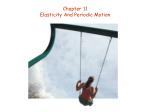Chapter 11

Elasticity And Periodic Motion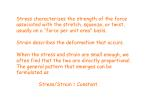Stress characterizes the strength of the force associated with the stretch, squeeze, or twist, usually on a “force per unit area” basis.

Strain describes the deformation that occurs.

When the stress and strain are small enough, we often find that the two are directly proportional. The general pattern that emerges can be formulated as

Stress/Strain = Constant.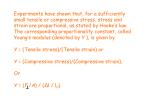Experiments have shown that, for a sufficiently small tensile or compressive stress, stress and strain are proportional, as stated by Hooke’s law. The corresponding proportionality constant, called Young’s modulus (denoted by Y ), is given by

Y = (Tensile stress)/(Tensile strain) or

Y = (Compressive stress)/(Compressive strain),

Or

Y = (F /A) / (Δl / l0)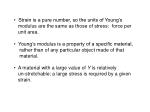Strain is a pure number, so the units of Young’s

• modulus are the same as those of stress: force per
• unit area.
• Young’s modulus is a property of a specific material,
• rather than of any particular object made of that
• material.
• A material with a large value of Y is relatively
• un-stretchable; a large stress is required by a given
• strain.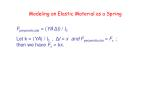Modeling an Elastic Material as a Spring

Fperpendicular = (YAΔl) / l0

Let k = (YA)/ l0 , Δl = x and Fperpendicular = Fx ; then we have Fx = kx.The pressure in a fluid, denoted by p, is the force Fperpendicular per unit area A transmitted across any cross section of the fluid, against a wall of its container, or against a surface of an immersed object:

p = Fperpendicular/A

When a solid object is immersed in a fluid and both are at rest, the forces that the fluid exerts on the surface of the object are always perpendicular to the surface at each point.When Hooke’s law is obeyed, the volume strain is proportional to the volume stress (change in pressure). The corresponding constant ratio of stress to strain is called the bulk modulus, denoted by B.

When the pressure on an object changes by a small amount Δp, from p0 to p0 + Δp, and the resulting volume strain is ΔV/V, Hooke’s law takes the form

B = - (Δp)/(ΔV/V0)

We include a minus sign in this equation because an increase in pressure always causes a decrease in volume. In other words, when Δp is positive, ΔV is negative.If the forces are small enough so that Hooke’s law is obeyed, the shear strain is proportional to the shear stress. The corresponding proportionality constant (ratio of shear stress to shear strain), is called the shear modulus, denoted by S:

S = Shear stress / Shear strain

= (Fparallel / A) / (x / h)

= (Fparallel / A) / ΦFx = -kx

Using Newton’s second law,

max = -kx

OR

ax = -(k/m)xAmplitude, A

• Cycle
• Period, T
• Frequency, f
• f = 1/T
• SI unit : Hertz (Hz) = cycle/s = 1/s
• Angular frequency, ω
• ω = 2πf = 2π/TEnergy in Simple Harmonic Motion

Conservation of Mechanical Energy

E = (1/2) mvx2 + (1/2)kx2 = constantA useful equation

When x = ± A, vx = 0. At this point, the energy is entirely potential energy and E = (1/2)kA2 .

E = (1/2)kA2 = (1/2) mvx2 + (1/2)kx2

vx = ± k/mA2 – x2

We can use this equation to find the magnitude of the velocity for any given position x.Position of the Shadow as a Function of Time

x = A cos θ

x = A cos(ωt)

x = A cos [(2π/T )t]

SI unit: mVelocity in Simple Harmonic Motion

v = -Aω sin(ωt)

SI unit: m/s

Maximum speed of the mass is

vmax = AωPeriod of a Mass on a Spring

From equation ax = -Aω2 cos(ωt)

ax = -Aω2 (maximum acceleration) (1)

Also, we know that

ax = -(kx)/m

ax = -(kA)/m (maximum acceleration)(2)

From equations (1) and (2)

-Aω2 = -kx/m

ω2 = k/m

ω= k/m = 2πf = 2π/T

T = 2πm / k

SI unit: sThe restoring force F at each point is the component of force tangent to the circular path at that point:

F = -mgsinθ

If the angle is small, sinθis very nearly equal toθ(in radians).

F = -mgθ = -mgx/L

F = -(mg/L)x

The restoring force F is then proportional to the coordinate x for small displacements, and the constant mg/L represents the force constant k.

K=mg/LNOTE that the frequency does NOT depend

on the mass but on the length of the pendulumA 0.85-kg mass attached to a vertical spring of force constant 150 N/m oscillates with a maximum speed of 0.35 m / s. Find the following quantities related to the motion of the mass: (a) the period, (b) the amplitude, (c) the maximum magnitude of the acceleration.A peg on a turntable moves with a constant linear speed of 0.67 m / s in a circle of radius 0.45 m. The peg casts a shadow on a wall. Find the following quantities related to the motion of the shadow: (a) the period, (b) the amplitude, (c) the maximum speed, and the maximum magnitude of acceleration.Ch. 11, Problem 42.

• An object of unknown mass is attached to an ideal spring with force constant 120 N/m and is found to vibrate with frequency of 6.00 Hz. Find
• period,
• the angular frequency, and
• the mass of this object.Ch. 11, Problem 49

• If a pendulum has period T and you double its
• length, what is its new period in terms of T?
• (b)If a pendulum has length L and you want to triple its frequency, what should be its length in terms of L?
• (c)Suppose a pendulum has a length L and period T on earth. If you take it to a planet where the acceleration of freely falling objects is ten times what it is on earth, what should you do to the length to keep the period the same as on earth?(d) If you do not change the pendulum’s length in part (c ), what is its period on that planet in terms of T?

(e) If a pendulum has a period T and you triple the mass of its bob, what happens to the period (in terms of T)?CHAPTER 11, PROBLEM 24

Find the period, frequency, and angular frequency of (a) the second hand and (b) the minute hand of a wall clock.

CHAPTER 11, Problem 47

A certain simple pendulum has a period on earth of 1.60 s. What is the period on the surface on Mars, where the acceleration due to gravity is 3.71 m/s2 ?Chapter 11, Problem 33

• A mass is oscillating with amplitude A at the end of a spring. How far (in terms of A) is this mass from equilibrium position of the spring when the elastic potential energy equals the kinetic energy?
• Chapter 11, Problem 34
• If a vibrating system has total energy E0, what will its total energy be (in terms of E0) if you double the amplitude of vibration?
• If you want to triple the total energy of a vibrating system with amplitude A0, what should its new amplitude be (in terms of A0)?Chapter 11, Problem 58

An object suspended from a spring vibrates with simple harmonic motion. At an instant when the displacement of the object is equal to one-half the amplitude, what fraction of the total energy of the system is kinetic and what fraction is potential?After you pick up a spare, your bowling ball rolls without slipping back toward the ball rack with a linear speed of 2.85 m/s. To reach the rack, the ball rolls up a ramp that rises through a vertical distance of 0.53 m. (a) What is the linear speed of the ball when it reaches the top of the ramp? (b) If the radius of the ball were increased, would the speed found in part (a) increase, decrease, or stay the same? Explain.CHAPTER 12

MECHANICAL WAVES AND SOUNDA disturbance that propagates from one place to another is referred to as a wave.

Waves propagate with well-defined speeds determined by the properties of the material through which they travel.

Waves carry energy.In a transverse wave individual particles move at right angles to the direction of wave propagation.

In a longitudinal wave individual particles move in the same direction as the wave propagation.As a wave on a string moves horizontally, all points on the string vibrate in the vertical direction.Sound Waves

Speed of Sound in Air

v = 343 m/sThe frequency of sound determines its pitch. High-pitched sounds have high frequencies; low-pitched sounds have low frequencies.

Human hearing extends from 20 Hz to 20, 000 Hz. Sounds with frequencies above this range are referred to as ultrasonic, while those with frequencies lower than 20 Hz are classified as infrasonic.In phase/opposite phase: Two sources are in phase if they both emit crests at the same time. Sources have opposite phase if one emits a crest at the same time other emits a trough.

Constructive interference occurs when the path length from the two sources differs by 0, λ, 2λ, 3λ, …….

Destructive interference occurs when the path length from the two sources differs by λ/2, 3λ/2, 5λ/2, …….The loudness of a sound is determined by its intensity; that is; by the amount of energy that passes through a given area in a given time.

I = E/(At)

Or

I = P/AI1 = P/(4πr12)

I2 = P/(4πr22)Chapter 3, Problem 50

• A cart carrying a vertical missile launcher moves horizontally at a constant velocity of 30.0 m/s to the right. It launches a rocket vertically upward. The missile has an initial vertical velocity of 40.0 m/s relative to the cart.
• How high does the rocket go?
• (b) How far does the cart travel
• while the rocket is in air?
• (c) Where does the rocket land
• relative to the cart?Chapter 3, Problem 53

• Your spaceship lands on an unknown planet. To determine the local value of g, you ask a steel-toed crew member to kick a stone, and you find that if she kicks it at 17.6 m/s at various angles, the maximum range she can achieve is 33.8 m.
• What is g on this planet?
• How long was the stone in the air?
• (c) How high above the ground did the stone go?Chapter 3 Problem 55

• A baseball thrown at an angle of 60.0° above the horizontal strikes a building 18.0 m away at a point 8.00 m above the point from which it was thrown. Ignore air resistance.
• Find the magnitude of the initial velocity of the
• baseball (the velocity with which the baseball is
• thrown).
• (b) Find the magnitude and direction of the velocity
• of the baseball just before it strikes the building.Chapter 11, Problem 30

• A 2.00 kg frictionless block is attached to an ideal spring with force constant 315 N/m. Initially the spring is neither stretched nor compressed, but the block is moving in the negative direction at 12.0 m/s. Find
• the amplitude of the motion,
• (b) the maximum acceleration of the block, and
• (c) the maximum force the spring exerts on the block.Chapter 11, Problem 31

• Repeat the previous problem, but assume that initially the block has velocity -4.00 m/s and displacement +0.200 m.
• Chapter 11, Problem 32
• A tuning fork has the tip of each of its two prongs vibrating in simple harmonic motion with a frequency of 392 Hz and an amplitude of 0.600 mm, while a housefly with mass 0.0270 g is holding onto the tip of one of the prongs. What are
• the maximum speed of the tip of a prong and
• (b) the fly’s maximum kinetic energy?